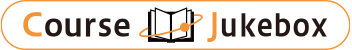### CourseDetailDegree
Bachelor
2
Course delivery methods
face-to-face
Subject
Social studies, Languages
Program
School
School of Social and International Studies
Department
College of International Studies
Campus
Tsukuba Campus
Classroom
3A409
Course Offering Year
2023-2024
Course Offering Month
October - December
Weekday and Period
Tue1,2
Capacity
Credits
2.0
Language
English
Course Number
BE22321

### Statistics University of Tsukuba

#### Course Overview

This course is a formal introduction to Statistics. No prior knowledge of probability and statistics is required as all concepts will be developed from the ground up. We will cover a range of topics including descriptive statistics, basics of probability, random variables, distribution and density functions, sampling distributions, point estimation, confidence intervals, and hypothesis testing. If time allows, a preview of the regression analysis will be provided. The details of regression analysis will be covered in Introductory Econometrics, which is a continuation of this course.

#### Learning Achievement

Students are expected to understand fundamental concepts of statistical inference and to apply statistical methods to simple exercises.

#### Competence

This course is a formal introduction to Statistics. No prior knowledge of probability and statistics is required. We will cover a range of topics including descriptive statistics, basics of probability, random variables, distribution and density functions, sampling distributions, point estimation, confidence intervals, and hypothesis testing. Regression analysis will be covered in Introductory Econometrics, which is a continuation of this course.

1. General-propose competence: critical and creative thinking skills, data and information literacy.
2. Special competence: analytical skills on international relations, analytical skills on international development.

#### Course prerequisites

No Prerequisites

Assignments(30%), Midterm Exam(30%) and Final Exam(40%)
Note that during the COVID-19 pandemic, the grading scheme is assignments (50%) and a take-home final exam (50%)

#### Course schedule

The course usually contains 9 lectures and 2 exams. Note that during the COVID-19 pandemic, we have 10 lectures and 1 take-home exam instead.
Introduction and descriptive statistics
Basics of probability
Random variables, cumulative distribution functions, discrete random variables
Continuous random variables, probability density function, Normal distribution
Two random variables and the joint distribution, covariance and correlation
Midterm exam
Chi-square distribution, Student's t distribution, F distribution, distributions of the sample mean and variance for normal populations. Large samples: law of large numbers and central limit theorem
Point estimation
Confidence intervals
Hypothesis testing
Not applicable

Lectures

YU ZHENGFEI

#### Other information

1. This course is taught online with asynchronous lecture sessions and synchronous Q&A sessions.

2. Regression analysis will be covered in Introductory Econometrics, which is a continuation of this course.

#### Site for Inquiry

Link to the syllabus provided by the university